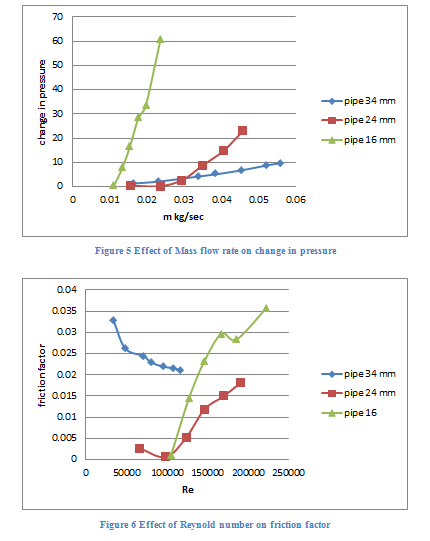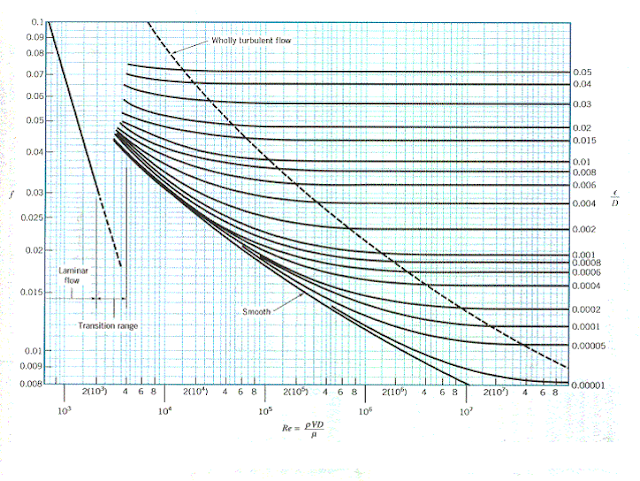# Flow of a Compressible Fluid Lab Report

Aim of this lab work is to understand the flow of a compressible fluid inside a tube

Following are some of the objectives which will lead to completion of above mention aim

1. Study the basic fluids, types of fluids and types of flow of fluid

2. Perform the numerical analysis to predict the flow of fluid in smooth pipe

3. Perform experiment of air flowing in smooth pipe

4. Calculate the Reynold number, friction factor and head losses in experiment

5. Compare the experimental result with calculated and numerical analysis

## Introduction on Flow of a Compressible Fluid

Fluid is anything which can flow between points of high potential to the point of low potential is called fluid. Ability of the fluid to flow is called its fluidity of fluid and it depends on the viscosity of the fluid.

Viscosity of the fluid is much like the density of the solid and it define the weight of the fluid per cubic meter. Higher the viscosity of fluid is higher will its weight per cubic meter and lower will be its fluidity.

Fluids can be classified in many different types based on the things under discussion for example discussing the Newton law fluids can be classified as Newtonian fluid and Non-Newtonian fluid.

Newtonian fluids are those fluids in which stresses at every point of flow are directly proportional and linear to strain and Non-Newtonian fluid are those fluids win which stresses at every point of flow are not directly proportional and linear to strain.

Based on the ability to compress fluids can be classified as compressible and non-compressible fluids.

Compressible fluid has the ability to get compress under high pressure means their particles just their position under pressure and non-compressible fluid are those fluid which does not show any change under pressure means their particle does not change their position under pressure.

### Types of fluid flow

There are three main types of fluid flow and all of them are based on the Reynold number of that particular fluid flowing at any particular speed. Reynold number is a dimension less number which shows the fluid inertial forces as compared to the viscous forces.

Based on the Reynold number fluid flow has three types; one is Laminar flow in which the Reynold number of the fluid never exceed 2100 value, transient flow is one in which Reynold number fluid flow lies between 2100 and 4000 value. Turbulent flow in one in which Reynold number of fluid flow exceeds 4000 value.

## Numerical Analysis

In order to predict the flow of fluid in any condition numerical analysis of that condition is done. Numerical analysis is a theoretical solution or the answer of the question related to the flow of fluid.

Computational fluid mechanics is used to predict the flow of fluid in any required conditions and as this process is very complex computer software like ansys is used to solve the equations involve in this process.

In order to perform the numerical analysis of this current process first the component involved in this work are needed to be modelled and for this any cad software or work bench of ansys can be used.

Figure one shown below is computer added model of pipe which represents the pipe used in this lab work. Second figure is of the fluid domain which represents the fluid which flow inside the pipe. Third figure represent the assembly of the pipe and fluid domain which will be used for analysis purpose.

In ansys the all components are first named as required like pipe, fluid domain, inlet and outlets and then they are meshed. Inlet of the pipe is on the right side of the pipe and outlet is on the left side (face visible in below figures).

In ansys setup the fluid domain is selected as fluid and air is selected as its material. For pipe material does not matter as long as analysis is limited to the flow of fluid. Pipe is made smooth by making the wall roughness zero in ansys setup.

In solution section transient solution will be done in order to simulate the flow of fluid inside the pipe with the initial velocity of fluid entering the pipe is as per experiment requirement.Result of the simulation shown above in figure 4 shows that at the inlet of the pipe the flow of fluid will be very turbulent as different velocities are visible in that region and along the length of the pipe the velocity on the top of the fluid domain and on the centre are different which shows fluid flow will be turbulent throughout the pipe.

Experimental Result
Table 1 Experimental valueThe friction factor mention in the above table is called the experimental frictional factor or the actual frictional factor as the data provided for its calculation was generated by performing an experiment.

Another way to get the friction factor is the moody chart which can predict the ideal friction factor based on the Reynold number and relative friction of surface.

According the calculation made based on the experimental data the frictional factor for 34 mm diameter pipe and initial velocity of 51.4 meter per second is 0.021.

According to the moody char for the Reynold number of 1.1*10^5 and smooth pipe the frictional factor is about 0.017 which is less than the experimental frictional factor.Figure 7 Moody Chart

Difference in the values of the experimental and graphs friction factor can be due to the fact that the pipe used in the experiment cannot be perfectly smooth that is the internal wall may have produce some extra frictional forces which result is additional pressure drop and increased value of frictional factor.

## Discussion on Flow of a Compressible Fluid

Numerical analysis of the said experiment was conducted using the computational fluid mechanics in ansys and result show that the flow of air inside the smooth pipe will be turbulent.

According to the experimental results the calculated Reynold number of the flow for all conditions is above 4000 mark which shows that the flow of air inside the pipe for experimental setup was turbulent.

So it can be concluded that the numerical analysis done for this experiment has successfully predicted the nature of flow of air inside the pipe.

In order observe the effect of mass flow rate on the change in pressure developed across the pipe, a graph was generated using the values obtain during the experiment. According to the graph the mass flow rate and change in pressure developed are directly proportional to each other.

This means that the increase of mass flow rate of air inside the pipe increase the pressure difference observed across the pipe and decrease of mass flow rate of air inside the pipe decreases the pressure difference observed across the pipe

In order observe the effect of Reynold number on the frictional factor, a graph was generated using the values obtain during the experiment. According to the graph the Reynold number and frictional factor are directly proportional to each other.

This means that the increase of Reynold number of air inside the pipe increase the frictional factor and decrease of Reynold Number of air inside the pipe decreases the Frictional factor for pipe with 24 mm and 16 mm diameter for 34 mm diameter this relation is opposite.